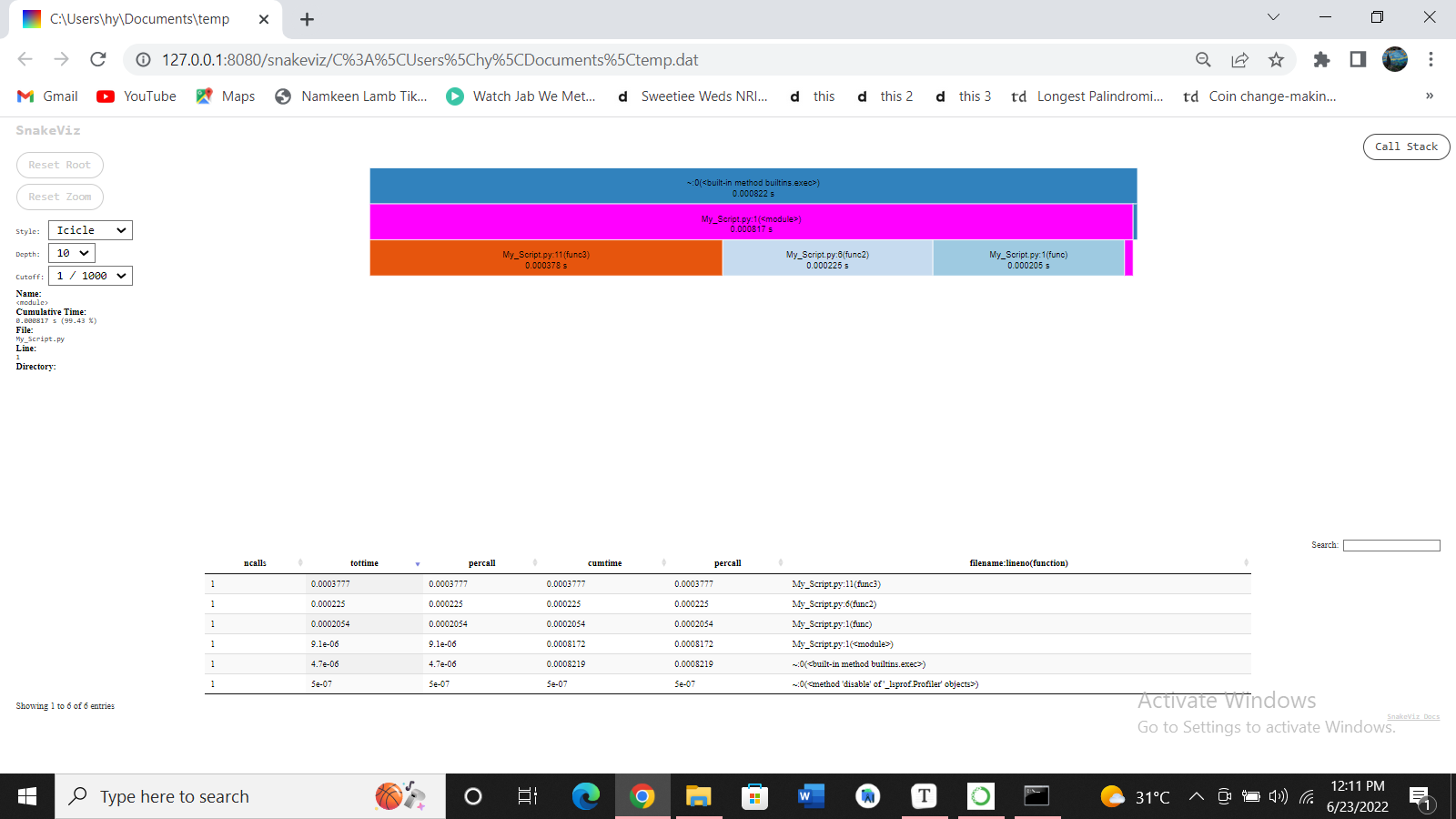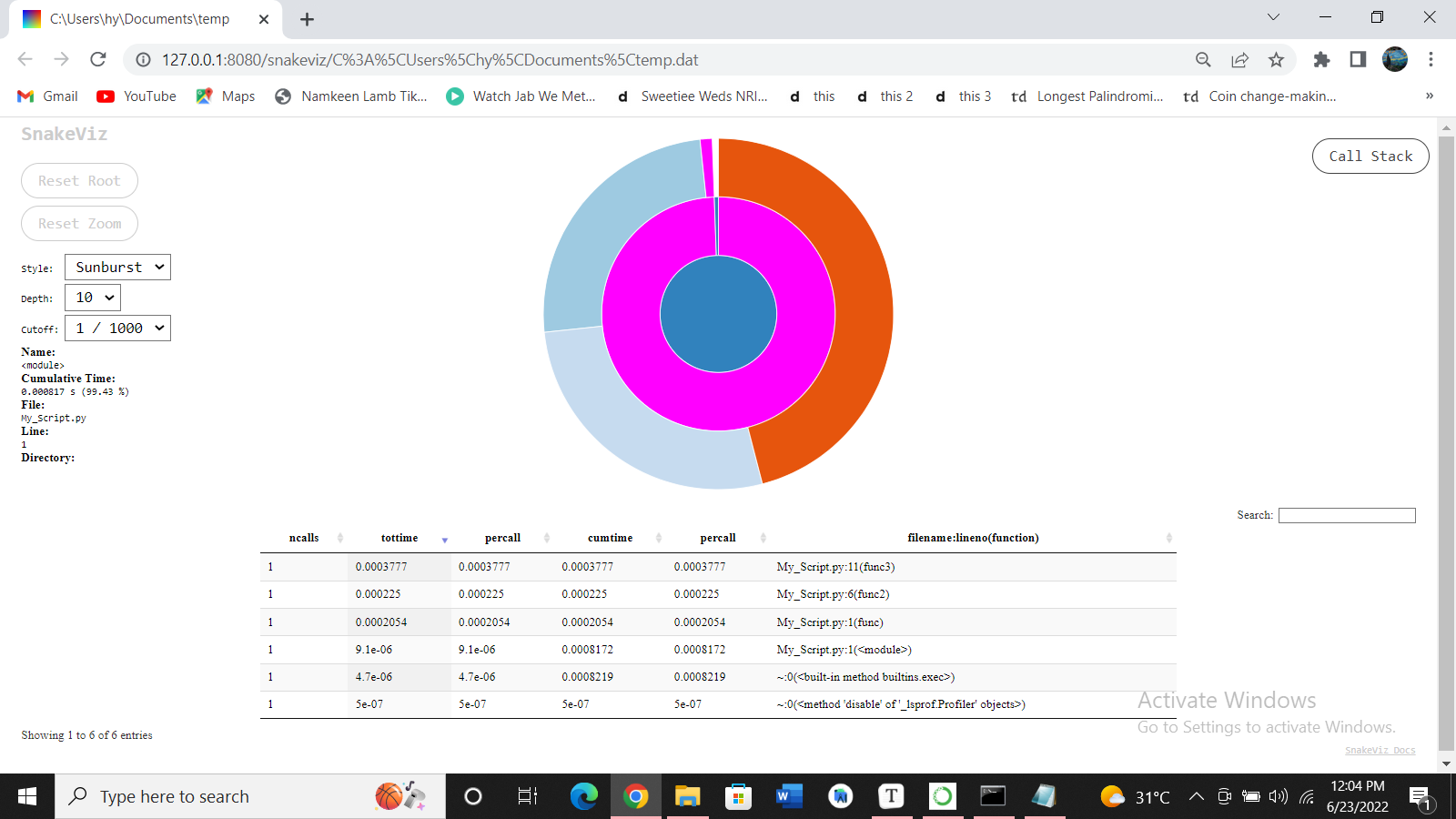# Profile a Python Code

Fariba Laiq Jul 01, 2022

Profiling is a technique that displays the statistics about how much time a script needs to execute and how often it executes. Through this, we can understand which part of the code needs to optimize and make the program efficient.

## Use `cProfile` to Profile a Python Code

`cProfile` is a built-in module in Python that measures the execution time of a program and also shows how often a method is called. To profile a Python code, we will write the code or the method name as a string argument in the `run()` method call.

Along with the total running time of the program, it also shows how much time a particular method takes to run.

In the following code, we have written a simple comparison operation as a string in the `run()` method call. The output shows all the statistics of that code.

Example Code:

``````import cProfile
cProfile.run("0 < 1")
``````

Output:

``````3 function calls in 0.000 seconds

Ordered by: standard name

ncalls  tottime  percall  cumtime  percall filename:lineno(function)
1    0.000    0.000    0.000    0.000 <string>:1(<module>)
1    0.000    0.000    0.000    0.000 {built-in method builtins.exec}
1    0.000    0.000    0.000    0.000 {method 'disable' of '_lsprof.Profiler' objects}
``````

Now, let’s write a code that took significant time with many method calls in the back end. In the following code, we have created a method containing nested loops that append the numbers to a list.

We will pass its name to the `run()` method to profile this method. The output shows all the statistics about this code.

Example Code:

``````import cProfile
def func():
num=[]
for i in range(1,100):
for j in range(1,100):
num.append(j)
cProfile.run("func()")
``````

Output:

``````9805 function calls in 0.009 seconds

Ordered by: standard name

ncalls  tottime  percall  cumtime  percall filename:lineno(function)
1    0.006    0.006    0.009    0.009 <ipython-input-3-d9419f0448d8>:2(func)
1    0.000    0.000    0.009    0.009 <string>:1(<module>)
1    0.000    0.000    0.009    0.009 {built-in method builtins.exec}
9801    0.003    0.000    0.003    0.000 {method 'append' of 'list' objects}
1    0.000    0.000    0.000    0.000 {method 'disable' of '_lsprof.Profiler' objects}
``````

## Use `SnakeViz` to Visualize the Profile Results in Python

`SnakeViz` is a web-based tool for profiling visualization. We can install and use it easily.

``````pip install snakeviz
``````

Suppose we want to profile and visualize the results of the following code. We have to save the code in a `py` file in Python’s environment.

We have three functions, each containing a loop that runs 1000 times and performs some arithmetic operations.

Example Code:

``````def func():
a=1
b=2
for i in range(1, 1000):
a += b
def func2():
a=1
b=2
for i in range(1, 1000):
a -= b
def func3():
a=1
b=2
for i in range(1, 1000):
a *= b
func()
func2()
func3()
``````

We will use the following commands to profile and visualize the results.

``````python -m cProfile -o temp.dat My_Script.py
``````
``````snakeviz temp.dat
``````

Output:`Icicle` (the default) and `Sunburst` are the two visualization modes offered by SnakeViz. In the case of Icicle, the width of a rectangle describes the amount of time spent in a function.

The arc’s angular extent represents the execution time if we switch to Sunburst. Using the `Style` option, we can change the two styles.

Author: Fariba Laiq

I am Fariba Laiq from Pakistan. An android app developer, technical content writer, and coding instructor. Writing has always been one of my passions. I love to learn, implement and convey my knowledge to others.